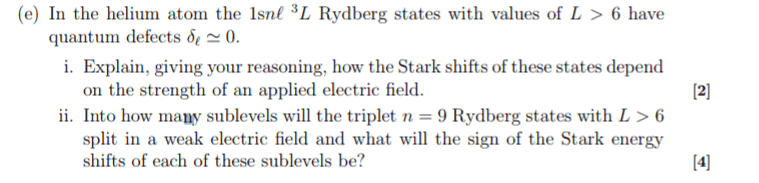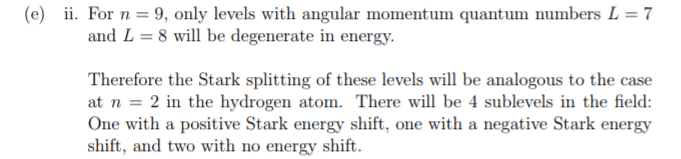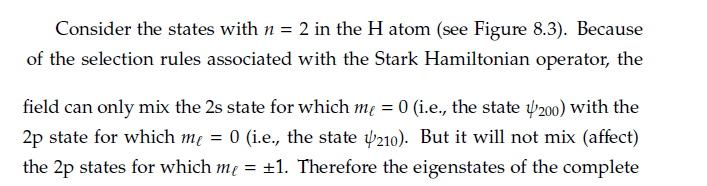# Qualitative question about the Stark effect

Clara Chung
Homework Statement:
Attached below
Relevant Equations:
Attached belowFor e ii) The answer isWhy are there only 4 sublevels?
We haven't learned about degenerate perturbation theory, the only thing mention in lecture iswhich I don't understand so I only memorize the good eigenfunctions for n=2. Could you explain why there are still only 4 sublevels for n=7 and 8?

Last edited by a moderator:

Homework Helper
2022 Award
which I don't understand so I only memorize the good eigenfunctions for n=2. Could you explain why there are still only 4 sublevels for n=7 and 8?
You do understand the Hydrogen atom Stark effect and not the Rydberg atom? (I'm not sure what you need here)...

•Clara Chung
Clara Chung
You do understand the Hydrogen atom Stark effect and not the Rydberg atom? (I'm not sure what you need here)...
For n=7 and n=8 there will be n^2 eigenfunction... if the states that will be mix are the states that satisfy the selection rules... i.e. delta l=+-1 and delta m=0, there will be so many... not only the two eigenstates in the hydrogen atom...?

Homework Helper
2022 Award
For n=7 and n=8 there will be n^2 eigenfunction... if the states that will be mix are the states that satisfy the selection rules... i.e. delta l=+-1 and delta m=0, there will be so many... not only the two eigenstates in the hydrogen atom...?
He is talking about the n=9 Rydberg states only. Within this are the allowed states L=0,1,...,8 each with multiplicity 2L+1. Only the highest angular momentum states are "far enough away" from the "nucleus/inner electron complex" to be approximately degenerate. So we are down to L=7,8. So how will this subset be affected by perturbation?

•Clara Chung
Clara Chung
He is talking about the n=9 Rydberg states only. Within this are the allowed states L=0,1,...,8 each with multiplicity 2L+1. Only the highest angular momentum states are "far enough away" from the "nucleus/inner electron complex" to be approximately degenerate. So we are down to L=7,8. So how will this subset be affected by perturbation?
If only L=7,8 are approximately degenerate... There are still many levels that can be mixed... Like l=7, ml=0,1,2,3,4,5,6,7 with l=8, ml=1,2,3,4,5,6,7...??

Homework Helper
2022 Award
If only L=7,8 are approximately degenerate... There are still many levels that can be mixed... Like l=7, ml=0,1,2,3,4,5,6,7 with l=8, ml=1,2,3,4,5,6,7...??
Yes all those states (let's be precise ml=0, ±1,..., ±L for L=7,8). But what about the (mixing) matrix elements. Are some zero? Are some the same size? This is not an easy exercise...but it is good. Clearly some levels will still be degenerate.

Clara Chung
Yes all those states (let's be precise ml=0, ±1,..., ±L for L=7,8). But what about the (mixing) matrix elements. Are some zero? Are some the same size? This is not an easy exercise...but it is good. Clearly some levels will still be degenerate.
How to know whether they have the same size? :(

Homework Helper
2022 Award
There are two states you know don't connect (create any off diagonal elements). What are they?

You say you have not had degenerate perturbation theory...why are you (we) looking at this problem? It is not obvious to me exactly how it simplifies completely as advertised.

Last edited:
•Clara Chung
Clara Chung
There are two states you know don't connect (create any off diagonal elements). What are they?

You say you have not had degenerate perturbation theory...why are you (we) looking at this problem? It is not obvious to me exactly how it simplifies completely as advertised.
Thank you for your help.. This appears in an exam of an introductory course on atomic physics... I guess I will leave it...